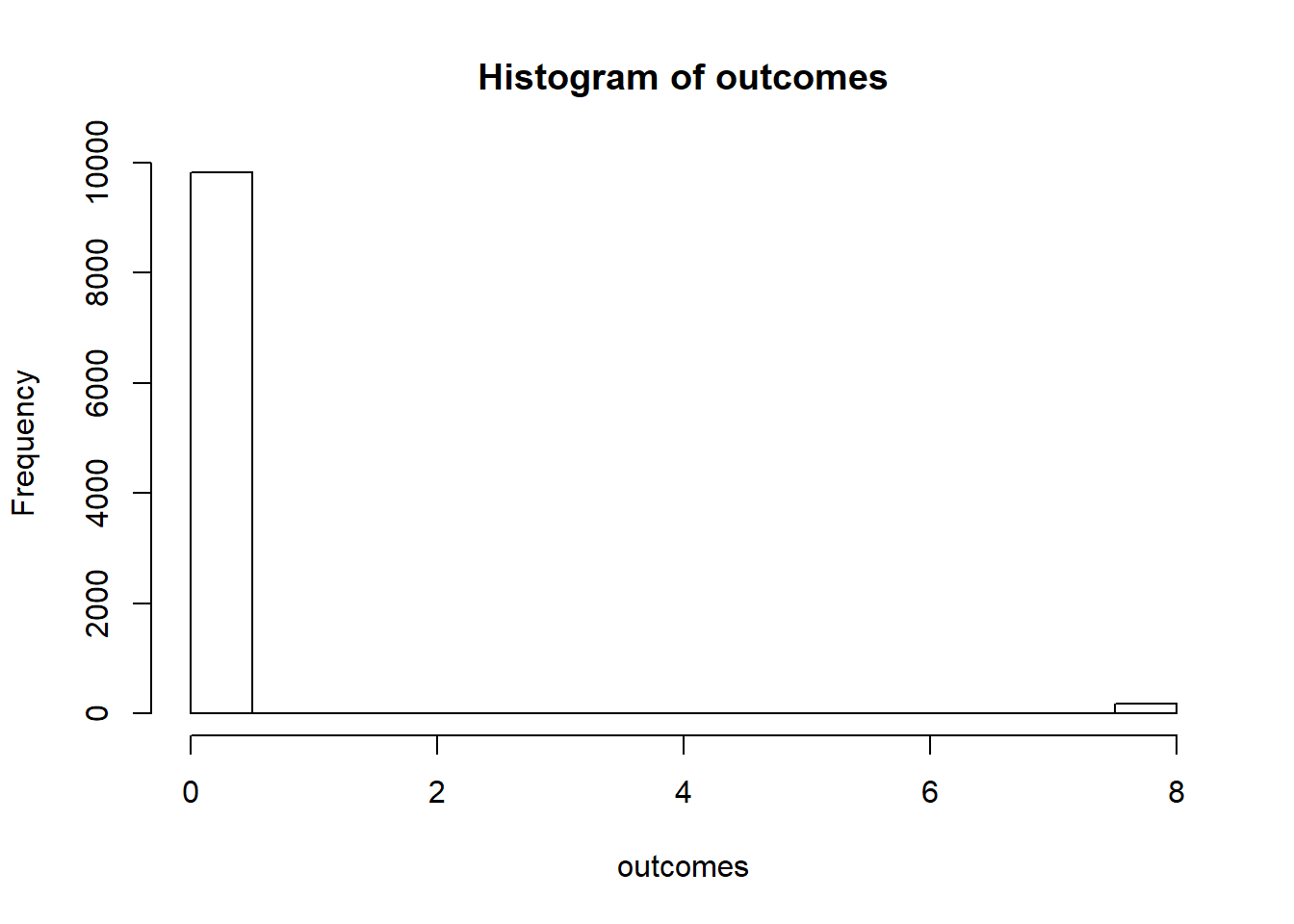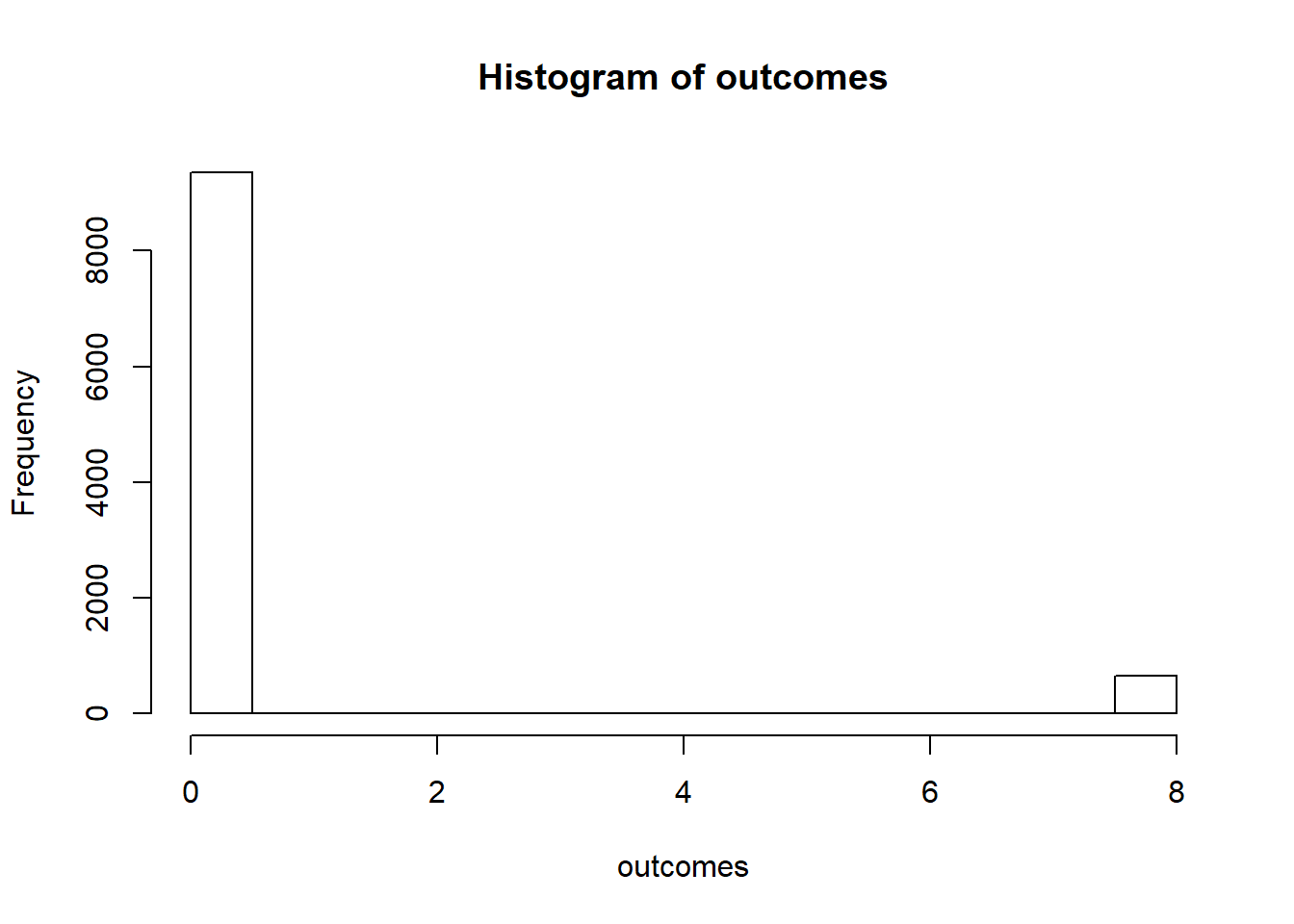# Probability - HW 10

## Question 1

Smith is in jail and has 1 dollar; he can get out on bail if he has 8 dollars. A guard agrees to make a series of bets with him.

If Smith bets A dollars, he wins A dollars with probability .4 and loses A dollars with probability .6. Find the probability that he wins 8 dollars before losing all of his money if

### Part A

1. he bets 1 dollar each time (timid strategy).

#### Theorem

$q_z = \frac{(q/p)^z - 1}{(q/p)^M-1}$ $q_z = \frac{(0.6/0.4)^1 - 1}{(0.6/0.4)^8-1}$

$q_1 = 0.0203$

#### Experimental

outcomes = c()
trials = 10000
for (i in (1:trials)){
money = 1
while (money > 0 && money < 8){
if(rbinom(1,1,0.4)==1){
money = money + 1
}
else {
money = money - 1
}
}
outcomes = append(outcomes, money)
}
hist(outcomes)print(paste('Experimental probability of winning with timid strategy:',  (sum(outcomes)/8)/trials))
##  "Experimental probability of winning with timid strategy: 0.0183"

### Part B

1. he bets, each time, as much as possible but not more than necessary to bring his fortune up to 8 dollars (bold strategy).

#### Experimental

outcomes = c()
trials = 10000
for (i in (1:trials)){
money = 1
while (money > 0 && money < 8){
if(rbinom(1,1,0.4)==1){
money = money + min(money, 8 - money)
}
else {
money = money - min(money, 8 - money)
}
}
outcomes = append(outcomes, money)
}
hist(outcomes)print(paste('Experimental probability of winning with timid strategy:',  (sum(outcomes)/8)/trials))
##  "Experimental probability of winning with timid strategy: 0.0652"

### Part C

1. Which strategy gives Smith the better chance of getting out of jail?

The bold strategy gives Smith the better chance of getting out of jail.